# Introduction to Data Structures

• Difficulty Level : Easy
• Last Updated : 04 Aug, 2022

WHAT IS DATA :

Data is the collection of different numbers, symbols, and alphabets to represent information.

WHAT IS DATA STRUCTURE :

A data structure is a group of data elements that provides the easiest way to store and perform different actions on the data of the computer. A data structure is a particular way of organizing data in a computer so that it can be used effectively. The idea is to reduce the space and time complexities of different tasks.

The choice of a good data structure makes it possible to perform a variety of critical operations effectively. An efficient data structure also uses minimum memory space and execution time to process the structure.

A data structure has also defined an instance of ADT.ADT means ABSTRACT DATA TYPE. It is formally defined as a triplet.[ D,F,A]

• D: Set of the domain.
• F:  the set of operations.
• A:  the set of axioms.

Type of data structure :

1.     Linear Data Structure
2.     Non-Linear Data Structure.

Linear Data Structure:

•  Elements are arranged in one dimension ,also known as linear dimension.
•  Example: lists, stack, queue, etc.

Non-Linear Data Structure

•   Elements are arranged in one-many, many-one and many-many dimensions.
•  Example: tree, graph, table, etc.

Data structures are used in various fields such as:

• Operating system
• Graphics
• Computer Design
• Blockchain
• Genetics
• Image Processing
• Simulation etc.Below is an overview of some popular data structures:

1. Array: An array is a collection of data items stored at contiguous memory locations. The idea is to store multiple items of the same type together. This makes it easier to calculate the position of each element by simply adding an offset to a base value, i.e., the memory location of the first element of the array (generally denoted by the name of the array).2. Linked Lists: Like arrays, Linked List is a linear data structure. Unlike arrays, linked list elements are not stored at a contiguous location; the elements are linked using pointers.3.Stack: Stack is a linear data structure which follows a particular order in which the operations are performed. The order may be LIFO(Last In First Out) or FILO(First In Last Out). In stack, all insertion and deletion are permitted at only one end of the list.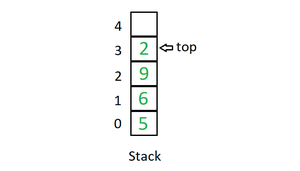Mainly the following three basic operations are performed in the stack:

• Initialize: Make a stack empty.
• Push: Adds an item in the stack. If the stack is full, then it is said to be an Overflow condition.
• Pop: Removes an item from the stack. The items are popped in the reversed order in which they are pushed. If the stack is empty, then it is said to be an Underflow condition.
• Peek or Top: Returns top element of the stack.
• isEmpty: Returns true if the stack is empty, else false.

4. Queue: Like Stack, Queue is a linear structure which follows a particular order in which the operations are performed. The order is First In First Out (FIFO). In the queue, items are inserted at one end and deleted from the other end. A good example of the queue is any queue of consumers for a resource where the consumer that came first is served first. The difference between stacks and queues is in removing. In a stack we remove the item the most recently added; in a queue, we remove the item the least recently added.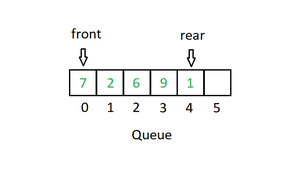Mainly the following four basic operations are performed on queue:

• Enqueue: Adds an item to the queue. If the queue is full, then it is said to be an Overflow condition.
• Dequeue: Removes an item from the queue. The items are popped in the same order in which they are pushed. If the queue is empty, then it is said to be an Underflow condition.
• Front: Get the front item from the queue.
• Rear: Get the last item from the queue.

5. Binary Tree: Unlike Arrays, Linked Lists, Stack and queues, which are linear data structures, trees are hierarchical data structures. A binary tree is a tree data structure in which each node has at most two children, which are referred to as the left child and the right child. It is implemented mainly using Links.

A Binary Tree is represented by a pointer to the topmost node in the tree. If the tree is empty, then the value of root is NULL. A Binary Tree node contains the following parts.

```1. Data
2. Pointer to left child
3. Pointer to the right child```

6.  Binary Search Tree: A Binary Search Tree is a Binary Tree following the additional properties:

• The left part of the root node contains keys less than the root node key.
• The right part of the root node contains keys greater than the root node key.
• There is no duplicate key present in the binary tree.

A Binary tree having the following properties is known as Binary search tree (BST).

7. Heap: A Heap is a special Tree-based data structure in which the tree is a complete binary tree. Generally, Heaps can be of two types:

• Max-Heap: In a Max-Heap the key present at the root node must be greatest among the keys present at all of its children. The same property must be recursively true for all sub-trees in that Binary Tree.
• Min-Heap: In a Min-Heap the key present at the root node must be minimum among the keys present at all of its children. The same property must be recursively true for all sub-trees in that Binary Tree.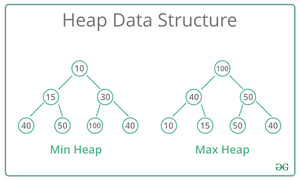8. Hashing Data Structure: Hashing is an important Data Structure which is designed to use a special function called the Hash function which is used to map a given value with a particular key for faster access of elements. The efficiency of mapping depends on the efficiency of the hash function used.

Let a hash function H(x) maps the value x at the index x%10 in an Array. For example, if the list of values is [11, 12, 13, 14, 15] it will be stored at positions {1, 2, 3, 4, 5} in the array or Hash table respectively.9. Matrix: A matrix represents a collection of numbers arranged in an order of rows and columns. It is necessary to enclose the elements of a matrix in parentheses or brackets.

A matrix with 9 elements is shown below.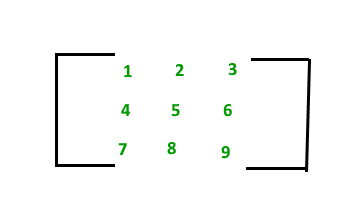10. Trie: Trie is an efficient information reTrieval data structure. Using Trie, search complexities can be brought to an optimal limit (key length). If we store keys in the binary search tree, a well-balanced BST will need time proportional to M * log N, where M is maximum string length and N is the number of keys in the tree. Using Trie, we can search the key in O(M) time. However, the penalty is on Trie storage requirements.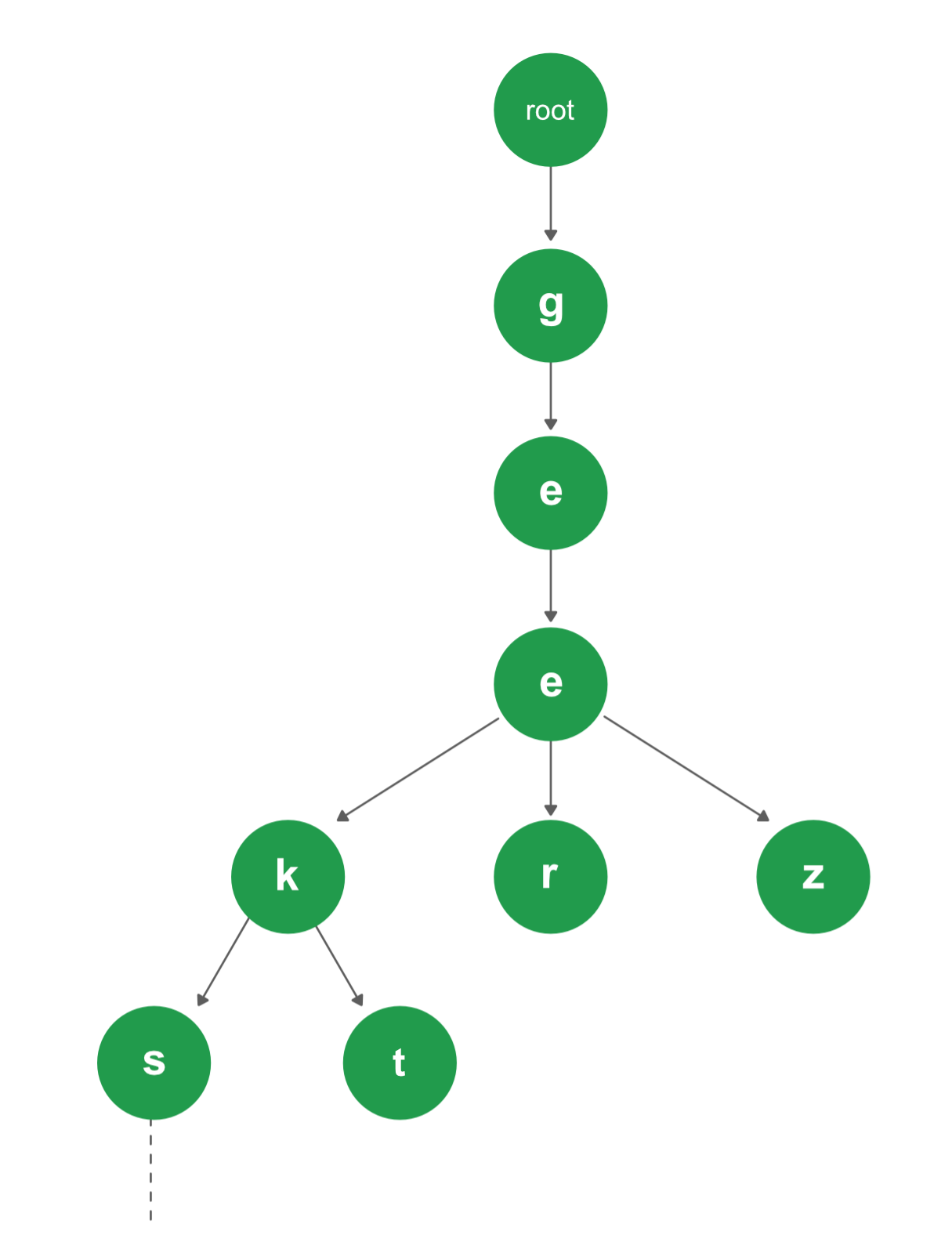My Personal Notes arrow_drop_up